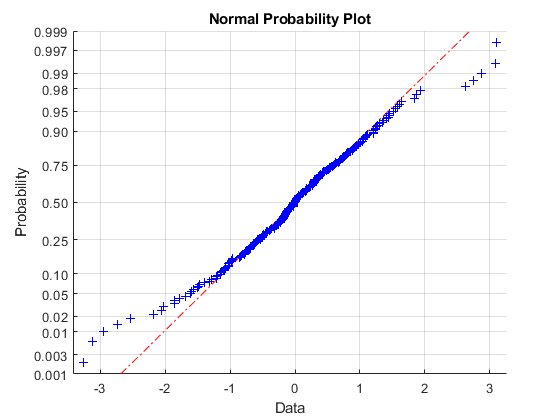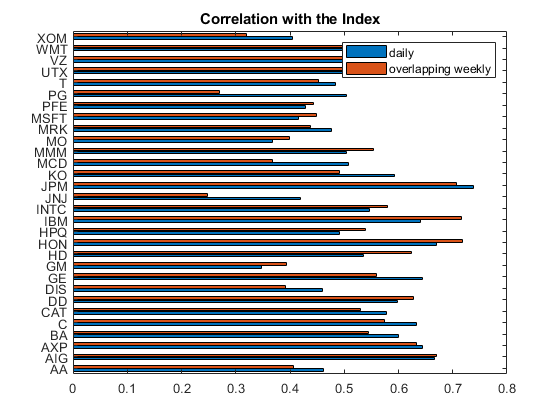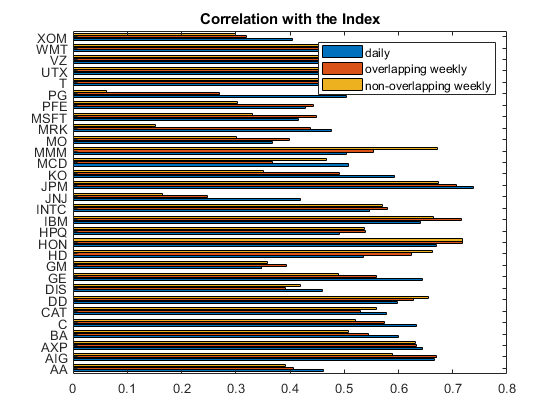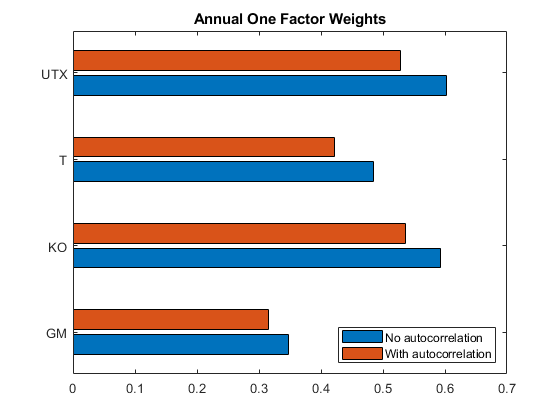Documentation

## One-Factor Model Calibration

This example demonstrates techniques to calibrate a one-factor model for estimating portfolio credit losses using the `creditDefaultCopula` or `creditMigrationCopula` classes.

This example uses equity return data as a proxy for credit fluctuations. With equity data, sensitivity to a single factor is estimated as a correlation between a stock and an index. The data set contains daily return data for a series of equities, but the one-factor model requires calibration on a year-over-year basis. Assuming that there is no autocorrelation, then the daily cross-correlation between a stock and the market index is equal to the annual cross-correlation. For stocks exhibiting autocorrelation, this example shows how to compute implied annual correlations incorporating the effect of autocorrelation.

### Fitting a One-Factor Model

Since corporate defaults are rare, it is common to use a proxy for creditworthiness when calibrating default models. The one-factor copula models the credit worthiness of a company using a latent variable, A:

`$A=wX+\sqrt{1-{w}^{2}}ϵ$`

where `X` is the systemic credit factor, `w` is the weight that defines the sensitivity of a company to the one factor, and $ϵ$ is the idiosyncratic factor. `w` and $ϵ$ have mean of 0 and variance of 1 and typically are assumed to be either Gaussian or else t distributions.

Compute the correlation between X and A:

`$Corr\left(A,X\right)=\frac{Cov\left(A,X\right)}{{\sigma }_{A}{\sigma }_{X}}$`

Since X and A have a variance of `1` by construction and $ϵ$ is uncorrelated with X, then:

`$\begin{array}{rl}Corr\left(A,X\right)& =Cov\left(A,X\right)=Cov\left(wX+\sqrt{1-{w}^{2}}ϵ,X\right)\\ & =wCov\left(X,X\right)+\sqrt{1-{w}^{2}}Cov\left(X,ϵ\right)=w\end{array}$`

If you use stock returns as a proxy for A and the market index returns are a proxy for X, then the weight parameter, w, is the correlation between the stock and the index.

### Prepare the Data

Use the returns of the Dow Jones Industrial Average (DJIA) as a signal for the overall credit movement of the market. The returns for the 30 component companies are used to calibrate the sensitivity of each company to the systemic credit movement. Weights for other companies in the stock market are estimated in the same way.

```% Read one year of DJIA price data t = readtable('dowPortfolio.xlsx'); % The table contains dates and the prices for each company at market close % as well as the DJIA. disp(head(t(:,1:7)))```
``` Dates DJI AA AIG AXP BA C _________ _____ _____ _____ _____ _____ _____ 1/3/2006 10847 28.72 68.41 51.53 68.63 45.26 1/4/2006 10880 28.89 68.51 51.03 69.34 44.42 1/5/2006 10882 29.12 68.6 51.57 68.53 44.65 1/6/2006 10959 29.02 68.89 51.75 67.57 44.65 1/9/2006 11012 29.37 68.57 53.04 67.01 44.43 1/10/2006 11012 28.44 69.18 52.88 67.33 44.57 1/11/2006 11043 28.05 69.6 52.59 68.3 44.98 1/12/2006 10962 27.68 69.04 52.6 67.9 45.02 ```
```% We separate the dates and the index from the table and compute daily returns using % tick2ret. dates = t{2:end,1}; index_adj_close = t{:,2}; stocks_adj_close = t{:,3:end}; index_returns = tick2ret(index_adj_close); stocks_returns = tick2ret(stocks_adj_close);```

### Compute Single Factor Weights

Compute the single-factor weights from the correlation coefficients between the index returns and the stock returns for each company.

```[C,daily_pval] = corr([index_returns stocks_returns]); w_daily = C(2:end,1);```

These values can be used directly when using a one-factor `creditDefaultCopula` or `creditMigrationCopula`.

Linear regression is often used in the context of factor models. For a one-factor model, a linear regression of the stock returns on the market returns is used by exploiting the fact that the correlation coefficient matches the square root of the coefficient of determination (R-squared) of a linear regression.

```w_daily_regress = zeros(30,1); for i = 1:30 lm = fitlm(index_returns,stocks_returns(:,i)); w_daily_regress(i) = sqrt(lm.Rsquared.Ordinary); end % The regressed R values are equal to the index cross correlations fprintf('Max Abs Diff : %e\n',max(abs(w_daily_regress(:) - w_daily(:))))```
```Max Abs Diff : 7.771561e-16 ```

This linear regression fits a model of the form $\mathit{A}=\alpha +\beta \text{\hspace{0.17em}}\mathit{X}+ϵ$, which in general does not match the one-factor model specifications. For example, $\mathit{A}$ and $\mathit{X}$ do not have a zero mean and a standard deviation of 1. In general, there is no relationship between the coefficient $\beta \text{\hspace{0.17em}}$ and the standard deviation of the error term $ϵ$. Linear regression is used above only as a tool to get the correlation coefficient between the variables given by the square root of the R-squared value.

For one-factor model calibration, a useful alternative is to fit a linear regression using the standardized stock and market return data $\stackrel{\sim }{\mathit{A}}$ and $\stackrel{\sim }{\mathit{X}}$. "Standardize" here means to subtract the mean and divide by the standard deviation. The model is $\stackrel{\sim }{\mathit{A}}=\stackrel{\sim }{\alpha }+\stackrel{\sim }{\beta }\text{\hspace{0.17em}}\stackrel{\sim }{\mathit{X}}+\stackrel{\sim }{ϵ}$. However, because both $\stackrel{\sim }{\mathit{A}}$ and $\stackrel{\sim }{\mathit{X}}$ have a zero mean, the intercept $\stackrel{\sim }{\alpha \text{\hspace{0.17em}}}$ is always zero, and because both $\stackrel{\sim }{\mathit{A}}$ and $\stackrel{\sim }{\mathit{X}}$ have standard deviation of 1, the standard deviation of the error term satisfies $\mathrm{std}\left(\stackrel{\sim }{ϵ\text{\hspace{0.17em}}}\right)=\sqrt{1-\stackrel{\sim }{{\beta \text{\hspace{0.17em}}}^{2}}}$. This exactly matches the specifications of the coefficients of a one-factor model. The one-factor parameter $\mathit{w}$ is set to the coefficient $\stackrel{\sim }{\beta \text{\hspace{0.17em}}}$, and is the same as the value found directly through correlation earlier.

```w_regress_std = zeros(30,1); index_returns_std = zscore(index_returns); stocks_returns_std = zscore(stocks_returns); for i = 1:30 lm = fitlm(index_returns_std,stocks_returns_std(:,i)); w_regress_std(i) = lm.Coefficients{'x1','Estimate'}; end % The regressed R values are equal to the index cross correlations fprintf('Max Abs Diff : %e\n',max(abs(w_regress_std(:) - w_daily(:))))```
```Max Abs Diff : 5.551115e-16 ```

This approach makes it natural to explore the distributional assumptions of the variables. The `creditDefaultCopula` and `creditMigrationCopula` objects support either normal distributions, or t distributions for the underlying variables. For example, when using `normplot` the market returns have heavy tails, therefore a t-copula is more consistent with the data.

`normplot(index_returns_std)`### Estimating Correlations for Longer Periods

The weights are computed based on the daily correlation between the stocks and the index. However, the usual goal is to estimate potential losses from credit defaults at some time further in the future, often one year out.

To that end, it is necessary to calibrate the weights such that they correspond to the one-year correlations. It is not practical to calibrate directly against historical annual return data since any reasonable data set does not have enough data to be statistically significant due to the sparsity of the data points.

You then face the problem of computing annual return correlation from a more frequently sampled data set, for example, daily returns. One approach to solving this problem is to use an overlapping window. This way you can consider the set of all overlapping periods of a given length.

```% As an example, consider an overlapping 1-week window. index_overlapping_returns = index_adj_close(6:end) ./ index_adj_close(1:end-5) - 1; stocks_overlapping_returns = stocks_adj_close(6:end,:) ./ stocks_adj_close(1:end-5,:) - 1; C = corr([index_overlapping_returns stocks_overlapping_returns]); w_weekly_overlapping = C(2:end,1); % Compare the correlation with the daily correlation. % Show the daily vs. the overlapping weekly correlations barh([w_daily w_weekly_overlapping]) yticks(1:30) yticklabels(t.Properties.VariableNames(3:end)) title('Correlation with the Index'); legend('daily','overlapping weekly');```The maximum cross-correlation p-value for daily returns show a strong statistical significance.

```maxdailypvalue = max(daily_pval(2:end,1)); disp(table(maxdailypvalue,... 'VariableNames',{'Daily'},... 'rownames',{'Maximum p-value'}))```
``` Daily __________ Maximum p-value 1.5383e-08 ```

Moving to an overlapping rolling-window-style weekly correlation gives slightly different correlations. This is a convenient way to estimate longer period correlations from daily data. However, the returns of adjacent overlapping windows are correlated so the corresponding p-values for the overlapping weekly returns are not valid since the p-value calculation in the `corr` function does not account for overlapping window data sets. For example, adjacent overlapping window returns are composed of many of the same datapoints. This tradeoff is necessary since moving to nonoverlapping windows could result is an unacceptably sparse sample.

```% Compare to non-overlapping weekly returns fridays = weekday(dates) == 6; index_weekly_close = index_adj_close(fridays); stocks_weekly_close = stocks_adj_close(fridays,:); index_weekly_returns = tick2ret(index_weekly_close); stocks_weekly_returns = tick2ret(stocks_weekly_close); [C,weekly_pval] = corr([index_weekly_returns stocks_weekly_returns]); w_weekly_nonoverlapping = C(2:end,1); maxweeklypvalue = max(weekly_pval(2:end,1)); % Compare the correlation with the daily and overlapping. barh([w_daily w_weekly_overlapping w_weekly_nonoverlapping]) yticks(1:30) yticklabels(t.Properties.VariableNames(3:end)) title('Correlation with the Index'); legend('daily','overlapping weekly','non-overlapping weekly');```The p-values for the nonoverlapping weekly correlations are much higher, indicating a loss of statistical significance.

```% Compute the number of samples in each series numDaily = numel(index_returns); numOverlapping = numel(index_overlapping_returns); numWeekly = numel(index_weekly_returns); disp(table([maxdailypvalue;numDaily],[NaN;numOverlapping],[maxweeklypvalue;numWeekly],... 'VariableNames',{'Daily','Overlapping','Non_Overlapping'},... 'rownames',{'Maximum p-value','Sample Size'}))```
``` Daily Overlapping Non_Overlapping __________ ___________ _______________ Maximum p-value 1.5383e-08 NaN 0.66625 Sample Size 250 246 50 ```

### Extrapolating Annual Correlation

A common assumption with financial data is that asset returns are temporally uncorrelated. That is, the asset return at time T is uncorrelated to the previous return at time T-1. Under this assumption, the annual cross-correlation is exactly equal to the daily cross-correlation.

Let ${X}_{t}$ be the daily log return of the market index on day t and ${A}_{t}$ be the daily return of a correlated asset. Using CAPM, the relation is modeled as:

`${A}_{t}=\alpha +\beta {X}_{t}+{ϵ}_{t}$`

The one-factor model is a special case of this relationship.

Under the assumption that asset and index returns are each uncorrelated with their respective past, then:

y, $\forall s\ne t:$

`$cov\left({X}_{s},{X}_{t}\right)=0$`

`$cov\left({ϵ}_{s},{ϵ}_{t}\right)=0$`

`$cov\left({A}_{s},{A}_{t}\right)=0$`

Let the aggregate annual (log) return for each series be

`$\underset{}{\overset{‾}{X}}=\sum _{t=1}^{T}{X}_{t}$`

`$\underset{}{\overset{‾}{A}}=\sum _{t=1}^{T}{A}_{t}$`

where T could be `252` depending on the underlying daily data.

Let ${\sigma }_{X}^{2}=var\left({X}_{t}\right)$ and ${\sigma }_{A}^{2}=var\left({A}_{t}\right)$ be the daily variances, which are estimated from the daily return data.

The daily covariance between ${X}_{t}$ and ${A}_{t}$ is:

`$cov\left({X}_{t},{A}_{t}\right)=cov\left({X}_{t},\alpha +\beta {X}_{t}+{ϵ}_{t}\right)=\beta {\sigma }_{X}^{2}$`

The daily correlation between ${X}_{t}$ and ${A}_{t}$ is:

`$corr\left({X}_{t},{A}_{t}\right)=\frac{cov\left({X}_{t},{A}_{t}\right)}{\sqrt{{\sigma }_{X}^{2}{\sigma }_{A}^{2}}}=\beta \frac{{\sigma }_{X}}{{\sigma }_{A}}$`

Consider the variances and covariances for the aggregate year of returns. Under the assumption of no autocorrelation:

`$var\left(\underset{}{\overset{‾}{X}}\right)=var\left(\sum _{t=1}^{T}{X}_{t}\right)=T{\sigma }_{X}^{2}$`

`$var\left(\underset{}{\overset{‾}{A}}\right)=var\left(\sum _{t=1}^{T}{A}_{t}\right)=T{\sigma }_{A}^{2}$`

`$cov\left(\underset{}{\overset{‾}{X}},\underset{}{\overset{‾}{A}}\right)=cov\left[\sum _{t=1}^{T}{X}_{t},\sum _{t=1}^{T}\left(\alpha +\beta {X}_{t}+{ϵ}_{t}\right)\right]=\beta cov\left(\underset{}{\overset{‾}{X}},\underset{}{\overset{‾}{X}}\right)=\beta var\left(\underset{}{\overset{‾}{X}}\right)=\beta T{\sigma }_{x}^{2}$`

The annual correlation between the asset and the index is:

`$corr\left(\underset{}{\overset{‾}{X}},\underset{}{\overset{‾}{A}}\right)=\frac{cov\left(\underset{}{\overset{‾}{X}},\underset{}{\overset{‾}{A}}\right)}{\sqrt{var\left(\underset{}{\overset{‾}{X}}\right)var\left(\underset{}{\overset{‾}{A}}\right)}}=\frac{\beta T{\sigma }_{X}^{2}}{\sqrt{T{\sigma }_{X}^{2}T{\sigma }_{A}^{2}}}=\beta \frac{{\sigma }_{X}}{{\sigma }_{A}}=w$`

Under the assumption of no autocorrelation, notice that the daily cross-correlation is in fact equal to the annual cross-correlation. You can use this assumption directly in the one-factor model by setting the one-factor weights to the daily cross-correlation.

### Handling Autocorrelation

If the assumption that assets have no autocorrelation is loosened, then the transformation from daily to annual cross-correlation between assets is not as straightforward. The $var\left(\underset{}{\overset{‾}{X\right)}}$ now has additional terms.

First consider the simplest case of computing the variance of $\underset{}{\overset{‾}{X}}$ when T is equal to `2`.

`$var\left(\underset{}{\overset{‾}{X}}\right)=\left[\begin{array}{cc}{\sigma }_{1}& {\sigma }_{2}\end{array}\right]\left[\begin{array}{cc}1& {\rho }_{12}\\ {\rho }_{12}& 1\end{array}\right]\left[\begin{array}{c}{\sigma }_{1}\\ {\sigma }_{2}\end{array}\right]={\sigma }_{1}^{2}+{\sigma }_{2}^{2}+2{\rho }_{12}{\sigma }_{1}{\sigma }_{2}$`

Since ${\sigma }_{1}={\sigma }_{2}={\sigma }_{X}$, then:

`$var\left(\underset{}{\overset{‾}{X}}\right)={\sigma }_{X}^{2}\left(2+2{\rho }_{12}\right)$`

Consider T = `3`. Indicate the correlation between daily returns that are $k$ days apart as ${\rho }_{\Delta k}$.

`$var\left(\underset{}{\overset{‾}{X}}\right)=\left[\begin{array}{ccc}{\sigma }_{1}& {\sigma }_{2}& {\sigma }_{3}\end{array}\right]\left[\begin{array}{ccc}1& {\rho }_{\Delta 1}& {\rho }_{\Delta 2}\\ {\rho }_{\Delta 1}& 1& {\rho }_{\Delta 1}\\ {\rho }_{\Delta 2}& {\rho }_{\Delta 1}& 1\end{array}\right]\left[\begin{array}{c}{\sigma }_{1}\\ {\sigma }_{2}\\ {\sigma }_{3}\end{array}\right]={\sigma }_{1}^{2}+{\sigma }_{2}^{2}+{\sigma }_{3}^{2}+2{\rho }_{\Delta 1}{\sigma }_{1}{\sigma }_{2}+2{\rho }_{\Delta 1}{\sigma }_{2}{\sigma }_{3}+2{\rho }_{\Delta 2}{\sigma }_{1}{\sigma }_{3}={\sigma }_{X}^{2}\left(3+4{\rho }_{\Delta 1}+2{\rho }_{\Delta 2}\right)$`

In the general case, for the variance of an aggregate T-day return with autocorrelation from trailing k days, there is:

`$var\left(\underset{}{\overset{‾}{X}}\right)=2{\sigma }_{X}^{2}\left(T/2+\left(T-1\right){\rho }_{\Delta 1}^{X}+\left(T-2\right){\rho }_{\Delta 2}^{X}+...+\left(T-k\right){\rho }_{\Delta k}^{X}\right)$`

This is also the same formula for the asset variance:

`$var\left(\underset{}{\overset{‾}{A}}\right)=2{\sigma }_{A}^{2}\left(T/2+\left(T-1\right){\rho }_{\Delta 1}^{A}+\left(T-2\right){\rho }_{\Delta 2}^{A}+...+\left(T-k\right){\rho }_{\Delta k}^{A}\right)$`

The covariance between $\underset{}{\overset{‾}{X}}$ and $\underset{}{\overset{‾}{A}}$ as shown earlier is equal to $\beta var\left(\underset{}{\overset{‾}{X}}\right)$.

Therefore, the cross-correlation between the index and the asset with autocorrelation from a trailing `1` through k days is:

`$corr\left(\underset{}{\overset{‾}{X}},\underset{}{\overset{‾}{A}}\right)=\frac{cov\left(\underset{}{\overset{‾}{X}},\underset{}{\overset{‾}{A}}\right)}{\sqrt{var\left(\underset{}{\overset{‾}{X}}\right)var\left(\underset{}{\overset{‾}{A}}\right)}}=\frac{\beta var\left(\underset{}{\overset{‾}{X}}\right)}{\sqrt{var\left(\underset{}{\overset{‾}{X}}\right)var\left(\underset{}{\overset{‾}{A}}\right)}}=\beta \sqrt{\frac{var\left(\underset{}{\overset{‾}{X}}\right)}{var\left(\underset{}{\overset{‾}{A}}\right)}}=...$`

`$corr\left(\underset{}{\overset{‾}{X}},\underset{}{\overset{‾}{A}}\right)=\beta \sqrt{\frac{2{\sigma }_{X}^{2}\left(T/2+\left(T-1\right){\rho }_{\Delta 1}^{X}+\left(T-2\right){\rho }_{\Delta 2}^{X}+...+\left(T-k\right){\rho }_{\Delta k}^{X}\right)}{2{\sigma }_{A}^{2}\left(T/2+\left(T-1\right){\rho }_{\Delta 1}^{A}+\left(T-2\right){\rho }_{\Delta 2}^{A}+...+\left(T-k\right){\rho }_{\Delta k}^{A}\right)}}$`

`$corr\left(\underset{}{\overset{‾}{X}},\underset{}{\overset{‾}{A}}\right)=\beta \frac{{\sigma }_{X}}{{\sigma }_{A}}\sqrt{\frac{T/2+\left(T-1\right){\rho }_{\Delta 1}^{X}+\left(T-2\right){\rho }_{\Delta 2}^{X}+...+\left(T-k\right){\rho }_{\Delta k}^{X}}{T/2+\left(T-1\right){\rho }_{\Delta 1}^{A}+\left(T-2\right){\rho }_{\Delta 2}^{A}+...+\left(T-k\right){\rho }_{\Delta k}^{A}}}$`

Note that $\beta \frac{{\sigma }_{X}}{{\sigma }_{A}}$ is the weight under the assumption of no autocorrelation. The square root term provides the adjustment to account for autocorrelation in the series. The adjustment depends more on the difference between the index autocorrelation and the stock autocorrelation, rather than the magnitudes of these autocorrelations. So the annual one-factor weight adjusted for autocorrelation is:

`${w}_{adjusted}=w\sqrt{\frac{T/2+\left(T-1\right){\rho }_{\Delta 1}^{X}+\left(T-2\right){\rho }_{\Delta 2}^{X}+...+\left(T-k\right){\rho }_{\Delta k}^{X}}{T/2+\left(T-1\right){\rho }_{\Delta 1}^{A}+\left(T-2\right){\rho }_{\Delta 2}^{A}+...+\left(T-k\right){\rho }_{\Delta k}^{A}}}$`

### Compute Weights with Autocorrelation

Look for autocorrelation in each of the stocks with the previous day's return, and adjust the weights to incorporate the effect of a one-day autocorrelation.

```corr1 = zeros(30,1); pv1 = zeros(30,1); for stockidx = 1:30 [corr1(stockidx),pv1(stockidx)] = corr(stocks_returns(2:end,stockidx),stocks_returns(1:end-1,stockidx)); end autocorrIdx = find(pv1 < 0.05)```
```autocorrIdx = 4×1 10 18 26 27 ```

There are four stocks with low p-values that may indicate the presence of autocorrelation. Estimate the annual cross-correlation with the index under this model, considering the one-day autocorrelation.

```% The weights based off of yearly cross correlation are equal to the daily cross % correlation multiplied by an additional factor. T = 252; w_yearly = w_daily; [rho_index, pval_index] = corr(index_returns(1:end-1),index_returns(2:end)); % Check to see if our index has any significant autocorrelation fprintf('One day autocorrelation in the index p-value: %f\n',pval_index);```
```One day autocorrelation in the index p-value: 0.670196 ```
```if pval_index < 0.05 % If the p-value indicates there is no significant autocorrelation in the index, % set its rho to 0. rho_index = 0; end w_yearly(autocorrIdx) = w_yearly(autocorrIdx) .*... sqrt((T/2 + (T-1) .* rho_index) ./ (T/2 + (T-1) .* corr1(autocorrIdx))); % Compare the adjusted annual cross correlation values to the daily values barh([w_daily(autocorrIdx) w_yearly(autocorrIdx)]) yticks(1:4); allNames = t.Properties.VariableNames(3:end); yticklabels(allNames(autocorrIdx)) title('Annual One Factor Weights'); legend('No autocorrelation','With autocorrelation','location','southeast');```